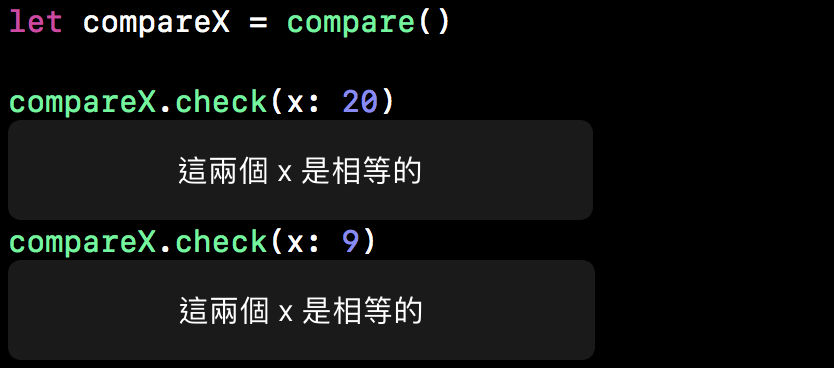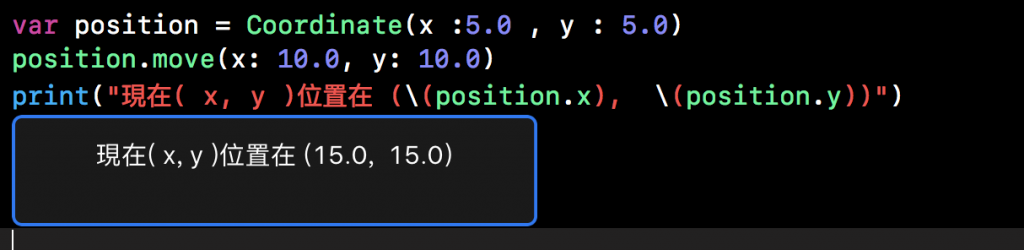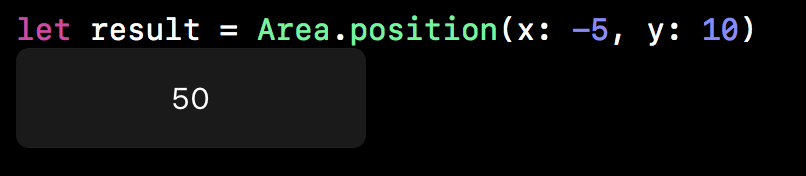#2018 iT 邦幫忙鐵人賽DAY 16
0
Software Development

# 方法 (Method)

``````class Clock {
var minute = 0
var hour = 0
func minCount(by min:Int) {
minute += min
}
func hrCount(by hr:Int) {
hour += hr
}
func minToHr() {
if minute >= 60 {
hour += minute / 60
minute -= 60 * (minute / 60)
}
}
}
``````

``````let timeSet = Clock()
timeSet.minCount(by: 241) // minute = 241
timeSet.hrCount(by: 5) // hour = 5
timeSet.minToHr() // minute 轉換為 hour
``````

# Self 屬性

`````` func minCount(by min:Int) {
self.minute += min
}
func hrCount(by hr:Int) {
self.hour += hr
}
``````

``````struct compare {
var x = 10
func check(x:Int) -> String {
var  msg = ""
if x > self.x {
msg = "你的輸入的 x 比我們的變數 x 還大"
} else {
msg = "你的輸入的 x 比我們的變數 x 還小"
}
return msg
}
}
````````````struct compare {
var x = 10
func check(x:Int) -> String {
var  msg = ""
if x == x {
msg = "這兩個 x 是相等的"
} else {
msg = "你的輸入的 x 比我們的變數 x 還小"
}
return msg
}
}
``````# 在實例方法中修改值類型

Struct 和 Enum 是值類型(value Type)。 默認情況下，值類型的屬性不能從其實例方法中修改。

``````struct Coordinate {
var x:Double  = 0.0 , y:Double = 0.0
mutating func move(x moveX:Double,y moveY:Double) {
x += moveX
y += moveY
}
}
``````

``````var position = Coordinate(x :5.0 , y : 5.0)
position.move(x: 10.0, y: 10.0)
``````# 在變異方法中指定自身

``````struct Coordinate {
var x:Double  = 0.0 , y:Double = 0.0
mutating func move(x moveX:Double,y moveY:Double) {
self = Coordinate(x: x + moveX , y: y + moveY)
}
}
``````

``````enum direction {
case up,down,left,righr
}
``````

``````enum direction {
case up, down, left, right
mutating func antiDirection(){
switch self{
case .up:
self = .down
case .down:
self = .up
case .left:
self = .right
case .right:
self = .left

}
}
}
``````

# 類型方法

``````class math {
func add(_ a:Int , _ b:Int) -> Int {
return a + b
}
}
let addResult = math()
print(addResult2.add(5, 10))
//print 15
``````

``````class math{
class func add(_ a:Int , _ b:Int) -> Int {
return a + b
}
}
let addResult = math.add(10, 5)
//addResult = 15
``````

``````struct Area {
static func position(x:Int , y:Int) -> Int {
var result = x * y
if result < 0 {
result = -result
return result
} else {
return result
}
}
}
``````Swift 菜鳥的30天30